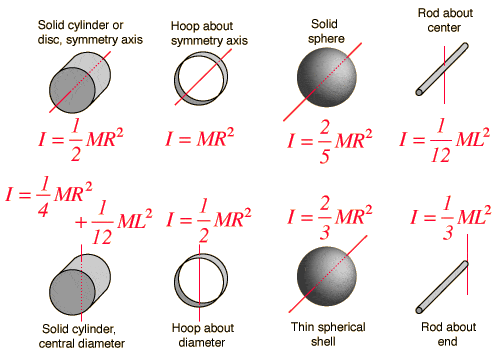# Physical pendulum formula

A rod suspended on its end and acting as a physical pendulum swings with a period of 1.74 s. What is its length? (g = 9.80 m/s2)

Ok so in order to solve this I need to use the physical pendulum formula:

T = 2Pi * sqrt (I / mgh) ==> I am looking for 'h'

To get 'h' I need to first calculate 'I' which requires mass for a rod.So, taking it from here I'm not sure where to begin. I've tried rearranging the formulas I've been given with no luck - any ideas?

## Answers and Replies

OlderDan
Science Advisor
Homework Helper
h is the distance from the pivot point to the center of mass of the rod. You can express that in terms of the length of the rod and you can express I in terms of the length of the rod.

Ok let me try this:
1.74=2Pi * sqrt ((1/12*mL^2) / (m*9.81*2L)
=> .27=sqrt((1/12L / 19.62))
1/12L = 1.504
L=~18m ??

This is wrong. I canceled out m and L in my early steps, did this throw my calculations off?

Doc Al
Mentor
The stick is suspended from its end, not its middle.

1.74=2Pi * sqrt((1/3L^2) / (m(9.81)(2L)

*question: I haven't had algebra for a while, but you can just cancel the m's and cancel the L's, leaving only one 'L' in the numerator, correct?

If that assumption is correct I get L=4.51m - my book disagrees with this answer though; any idea why?

Doc Al
Mentor
Looks like you are setting h = 2L, which isn't right. As OlderDan explained, h is the distance from the pivot point to the center of mass of the rod.